# Kinematics fundamentals by Sunil Singh - HTML preview

PLEASE NOTE: This is an HTML preview only and some elements such as links or page numbers may be incorrect.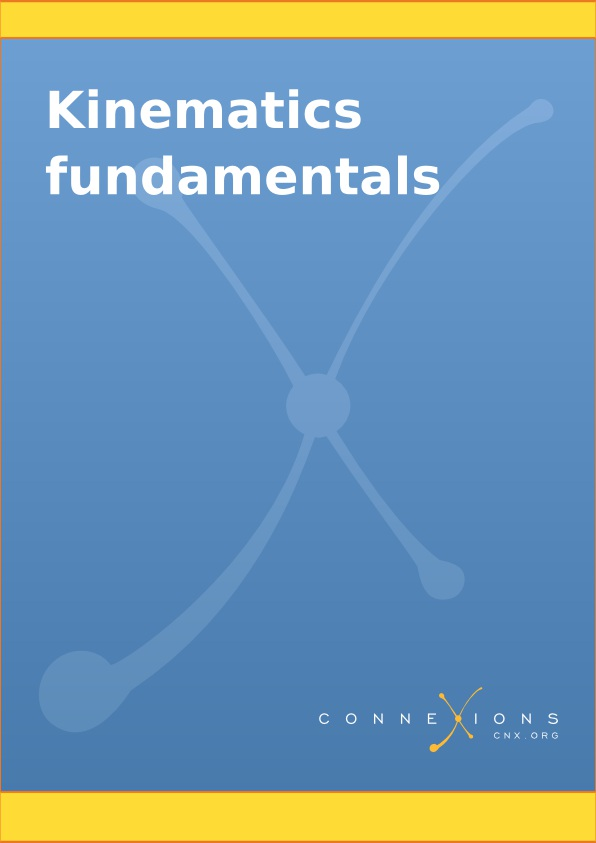Kinematics fundamentals

By: Sunil Singh

This selection and arrangement of content as a collection is copyrighted by Sunil Singh.

Collection structure revised: 2008/09/28

For copyright and attribution information for the modules contained in this collection, see the " Attributions" section at the end of the collection.

Kinematics fundamentals

Chapter 1. Motion

1.1. Motion

We require an observer to identify motion

Frame of reference and observer

We need to change our mind set

Is there an absolute frame of reference?

Motion types

Kinematics

1.2. Coordinate systems in physics

Coordinate system types

Rectangular (Cartesian) coordinate system

Spherical coordinate system

Cylindrical coordinate system

1.3. Distance

Distance – time plot

1.4. Position

Position

Plotting motion

Description of motion

Position – time plot

1.5. Vectors

What is a vector?

Vector algebra

Unit vector

Other important vector terms

Null vector

Negative vector

Co-planar vectors

Axial vector

Why should we study vectors?

Triangle law

Parallelogram law

Polygon law

Subtraction

Vector sum and difference

Lami's theorem

Exercises

1.7. Components of a vector

Components of a vector

Planar components of a vector

Representation of a vector in component form

Exercises

1.8. Scalar (dot) product

Multiplication with scalar

Products of vectors

Scalar product (dot product)

Angle between vectors

Meaning of scalar product

Values of scalar product

Scalar product in component form

Component as scalar (dot) product

Attributes of scalar (dot) product

Law of cosine and dot product

Differentiation and dot product

Exercises

1.9. Scalar product (application)

Representative problems and their solutions

Angle between two vectors

Condition of perpendicular vectors

Component as scalar product

Nature of scalar product

Scalar product of a vector with itself

Evaluation of dot product

1.10. Vector (cross) product

Direction of vector product

Values of cross product

Cross product in component form

Geometric meaning vector product

Attributes of vector (cross) product

1.11. Vector product (application)

Representative problems and their solutions

Condition of parallel vectors

Unit vector of cross product

Nature of vector product

Evaluation of vector product

Area of parallelogram

1.12. Position vector

Position Vector in component form

Motion types and position vector

Examples

1.13. Displacement

Displacement and Position vector

Displacement and dimension of motion

Displacement – time plot

Interpreting change of position

Example

1.14. Speed

Distance .vs. time plots

Average speed

Instantaneous speed (v)

Speed - time plot

Position - time plot

1.15. Velocity

Position vector and velocity

Average velocity

Position – time plot and average velocity

Instantaneous velocity

Instantaneous velocity and position - time plot

Components of velocity

Few words of caution

Summary

Exercises

1.16. Rectilinear motion

Position vector in rectilinear motion

Vector interpretation and equivalent system of scalars

Position - time plot

Nature of slope

Direction of motion

Variation in the velocity

Velocity - time plot

The nature of velocity – time plot

Area under velocity – time plot

Uniform motion

Motion of separated bodies

1.17. Rectilinear motion (application)

Representative problems and their solutions

Position vector

Interpretation of displacement - time plot

Displacement

Average velocity

1.18. Understanding motion

Similarities and differences

1.19. Velocity (application)

Representative problems and their solutions

Position vector

Displacement

Constrained motion

Nature of velocity

Comparing velocities

Chapter 2. Acceleration

2.1. Acceleration

Acceleration

Average acceleration

Instantaneous acceleration

Acceleration in terms of position vector

2.2. Understanding acceleration

Velocity, acceleration and force

External force and possible scenarios

2.3. Acceleration and deceleration

Negative vector quantities

“Increase” and “decrease” of vectors quantities

Deceleration

Graphical interpretation of negative vector quantities describing motion

Position vector

Velocity vector

Acceleration vector

2.4. Accelerated motion in one dimension

Nature of acceleration in one dimensional motion

Graphs of one dimensional motion

Acceleration – time plot

Area under acceleration - time plot

Velocity – time plot

2.5. Constant acceleration

Understanding constant acceleration

Equation of motion

First equation

Second equation

Third equation

Graphical interpretation of equations of motion

Equations of motion in component form

Equivalent scalar system of equations of motion

2.6. Constant acceleration (application)

Hints on solving problems

Representative problems and their solutions

Average velocity

Differentiation and Integration methods

Components of acceleration

Rectilinear motion with constant acceleration

Equations of motion

2.7. One dimensional motion with constant acceleration

Equation of motion for one dimensional motion with constant acceleration

Motion under gravity

Displacement in a particular second

Average acceleration

Interpretation of equations of motion

Constant acceleration (force) is applied in the direction of velocity

Constant acceleration (force) is applied in the opposite direction of velocity

Exercises

2.8. Graphs of motion with constant acceleration

Nature of graphs

Nature of coefficient of squared term

Nature of discriminant

Acceleration is positive (in the reference direction)

Acceleration is negative (opposite to the reference direction)

Example

2.9. Vertical motion under gravity

Velocity

Displacement and distance

Position

Exercises

2.10. Vertical motion under gravity (application)

Representative problems and their solutions

Motion plots

Equal displacement

Equal time

Displacement in a particular second

Twice in a position

Collision in air

2.11. Non-uniform acceleration

Important calculus results

Differentiation

Integration

Velocity and acceleration is expressed in terms of time “t

Velocity and acceleration is expressed in terms of time “x”

Acceleration in terms of velocity

Obtaining expression of velocity in time, “t”

Obtaining expression of velocity in time, “x”

Graphical method

Velocity .vs. time

Acceleration .vs. time

Exercises

Chapter 3. Relative motion

3.1. Relative velocity in one dimension

Relative motion in one dimension

Position of the point object

Velocity of the point object

Acceleration of the point object

Interpretation of the equation of relative velocity

Equation with reference to earth

Order of subscript

Evaluating relative velocity by making reference object stationary

Direction of relative velocities

Relative velocity .vs. difference in velocities

Relative acceleration

Worked out problems

3.2. Relative velocity in one dimension(application)

Hints on solving problems

Representative problems and their solutions

3.3. Relative velocity in two dimensions

Relative motion in two dimensions

Position of the point object

Velocity of the point object

Acceleration of the point object

Interpretation of the equation of relative velocity

Equation with reference to earth

Evaluation of equation using analytical technique

Equation in component form

3.4. Relative velocity in two dimensions (application)

Hints on solving problems

Representative problems and their solutions

Velocity of an individual object

Relative velocity

Closest approach

3.5. Analysing motion in a medium

Concept of independence of motion

Motion of boat in a stream

Resultant velocity

Time to cross the stream

Drift of the boat

Special cases

Shortest interval of time to cross the stream

Direction to reach opposite point of the stream

The velocity to reach opposite point of the stream

Shortest path

Motion of an object in a medium

3.6. Resultant motion (application)

Representative problems and their solutions

Velocity of the object

Time to cross the river

Multiple references

Minimum time, distance and speed

Chapter 4. Accelerated motion in two dimensions

4.1. Projectile motion

Projectile motion

Force(s) in projectile motion

Analysis of projectile motion

Projectile motion and equations of motion

Initial velocity

Equations of motion in vertical direction

Velocity

Displacement

Time of flight

Equations of motion in horizontal direction

Displacement of projectile

Velocity of projectile

Equation of the path of projectile

Exercises

Projectile motion (application)

Representative problems and their solutions

Direction of motion on return

Maximum height

Equation of projectile motion

Change in angles during motion

Kinetic energy of a projectile

Change in the direction of velocity vector

Features of projectile motion

Time of flight, T

Maximum height reached by the projectile, H

Range of projectile, R

Equation of projectile motion and range of projectile

Impact of air resistance

Situations involving projectile motion

Clearing posts of equal height

Hitting a specified target

Determining attributes of projectile trajectory

Exercises

More exercises

Features of projectile motion (application)

Hints on solving problems

Representative problems and their solutions

Time of flight

Horizontal range

Maximum height

Height attained by a projectile

Composition of motion

Projectile motion with wind/drag force

Projectile motion types

Projection from a higher level

Projectile thrown in horizontal direction

Projectile thrown at an angle with horizontal direction

Projectile thrown up at an angle with horizontal direction

Projectile thrown down at an angle with horizontal direction

Exercises

Acknowledgment

Projectile motion types (application)

Hints on solving problems

Representative problems and their solutions

Time of flight

Range of flight

Initial velocity

Final velocity

Projectile motion on an incline

Analyzing alternatives

Projection up the incline

Coordinates along incline (x) and perpendicular to incline (y)

Time of flight

Range of flight

Maximum range

Coordinates in horizontal (x) and vertical (y) directions

Projection down the incline

Exercises

Projectile motion on an incline (application)

Hints for solving problems

Representative problems and their solutions

Range of the flight

Angle of projection

Final speed of the projectile

Elastic collision with the incline

Projectile motion on two inclines

Relative motion of projectiles

Relative velocity of projectiles

Interpretation of relative velocity of projectiles

Relative velocity in horizontal direction

Relative velocity in vertical direction

Resultant relative motion

Physical interpretation of relative velocity of projectiles

Special cases

Exercises

Collision of projectiles

Analysis of motion

Relative motion in x-direction

Relative motion in y-direction

Collision of projectiles initiated without vertical separation

Collision of projectiles initiated without horizontal separation

Collision of projectiles initiated from different horizontal and vertical levels

Exercises

4.2. Circular motion

Uniform circular motion

Requirement of uniform circular motion

Analysis of uniform circular motion

Coordinates of the particle

Position of the particle

Velocity of the particle

Centripetal acceleration

Direction of centripetal acceleration

Time period of uniform circular motion

Uniform circular motion and projectile motion

Exercise

Uniform circular motion (application)

Representative problems and their solutions

Direction of velocity

Direction of position vector

Velocity

Relative speed

Nature of UCM

Circular motion and rotational kinematics

Angular quantities

Angular position (θ)

Angular displacement (Δθ)

Angular velocity (ω)

Description of circular motion

Relationship between linear (v) and angular speed (ω)

Vector representation of angular quantities

Linear and angular velocity relation in vector form

Uniform circular motion

Linear .vs. angular quantity

Exercises

Circular motion and rotational kinematics (application)

Representative problems and their solutions

Measurement of angular displacement

Angular speeds

Centripetal acceleration

Accelerated motion in two dimensions

Characteristics of two dimensional motion

Tangential acceleration

Normal acceleration

Total acceleration

Tangential and normal accelerations in circular motion

Elliptical motion

Path of motion

Nature of velocity and acceleration

Application

Representative problems and their solutions

Path of motion

Tangential and normal accelerations

Nature of motion

Displacement in two dimensions

Transformation of graphs

Important concepts

Graph of a function

Input to the function

Output of the function

Arithmetic operations

Product/division operations

Negation

Effect of arithmetic operations

Forms of representation

Transformation of graph by input

Addition and subtraction to independent variable

Multiplication and division of independent variable

Negation of independent variable

Combined input operations

Horizontal shift

Non-uniform circular motion

Angular velocity

Tangential acceleration

Angular acceleration

Relationship between linear and angular acceleration

Linear and angular acceleration relation in vector form

Uniform circular motion

Description of circular motion using vectors

Exercises

Non – uniform circular motion(application)

Hints on problem solving

Representative problems and their solutions

Velocity

Average total acceleration

Total acceleration

Circular motion with constant acceleration

What corresponds to what ?

Angular quantities

Basic equations

Derived equations

Sign of angular quantities

Angular velocity

Angular displacement

Exercises

Circular motion with constant acceleration (application)

Check understanding

Hints for problem solving

Representative problems and their solutions

Nature of angular motion

Time interval

Angular displacement

Index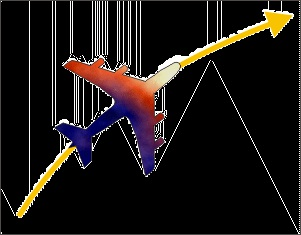Chapter 1. Motion

1.1. Motion*

Motion is a state, which indicates change of position. Surprisingly, everything in this world is

constantly moving and nothing is stationary. The apparent state of rest, as we shall learn, is a

notional experience confined to a particular system of reference. A building, for example, is at

rest in Earth’s reference, but it is a moving body for other moving systems like train, motor,

airplane, moon, sun etc.

Figure 1.1. Motion of an airplane

The position of plane with respect to the earth keeps changing with time.

Definition: Motion

Motion of a body refers to the change in its position with respect to time in a given frame of

reference.

A frame of reference is a mechanism to describe space from the perspective of an observer. In

other words, it is a system of measurement for locating positions of the bodies in space with

respect to an observer (reference). Since, frame of reference is a system of measurement of

positions in space as measured by the observer, frame of reference is said to be attached to the

observer. For this reason, terms “frame of reference” and “observer” are interchangeably used to

describe motion.

In our daily life, we recognize motion of an object with respect to ourselves and other stationary

objects. If the object maintains its position with respect to the stationary objects, we say that the

object is at rest; else the object is moving with respect to the stationary objects. Here, we conceive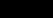all objects moving with earth without changing their positions on earth surface as stationary

objects in the earth’s frame of reference. Evidently, all bodies not changing position with respect

to a specific observer is stationary in the frame of reference attached with the observer.

We require an observer to identify motion

Motion has no meaning without a reference system.

An object or a body under motion, as a matter of fact, is incapable of identifying its own motion.

It would be surprising for some to know that we live on this earth in a so called stationary state

without ever being aware that we are moving around sun at a very high speed - at a speed faster

than the fastest airplane that the man kind has developed. The earth is moving around sun at a

speed of about 30 km/s (≈ 30000 m/s ≈ 100000 km/hr) – a speed about 1000 times greater than

the motoring speed and 100 times greater than the aircraft’s speed.

Likewise, when we travel on aircraft, we are hardly aware of the speed of the aircraft. The state of

fellow passengers and parts of the aircraft are all moving at the same speed, giving the impression

that passengers are simply sitting in a stationary cabin. The turbulence that the passengers

experience occasionally is a consequence of external force and is not indicative of the motion of

the aircraft.

It is the external objects and entities which indicate that aircraft is actually moving. It is the

passing clouds and changing landscape below, which make us think that aircraft is actually

moving. The very fact that we land at geographically distant location at the end of travel in a short

time, confirms that aircraft was actually cruising at a very high speed.

The requirement of an observer in both identifying and quantifying motion brings about new

dimensions to the understanding of motion. Notably, the motion of a body and its measurement is

found to be influenced by the state of motion of the observer itself and hence by the state of

motion of the attached frame of reference. As such, a given motion is evaluated differently by

different observers (system of references).

Two observers in the same state of motion, such as two persons standing on the platform, perceive

the motion of a passing train in exactly same manner. On the other hand, the passenger in a

speeding train finds that the other train crossing it on the parallel track in opposite direction has

the combined speed of the two trains

. The observer on the ground, however, find them

running at their individual speeds v 1 and v 2.

From the discussion above, it is clear that motion of an object is an attribute, which can not be

stated in absolute term; but it is a kind of attribute that results from the interaction of the motions

of the both object and observer (frame of reference).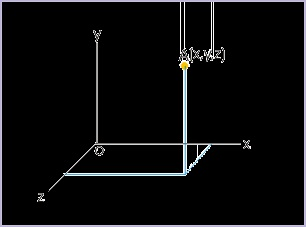Frame of reference and observer

Frame of reference is a mathematical construct to specify position or location of a point object in

space. Basically, frame of reference is a coordinate system. There are plenty of coordinate

systems in use, but the Cartesian coordinate system, comprising of three mutually perpendicular

axes, is most common. A point in three dimensional space is defined by three values of

coordinates i.e. x,y and z in the Cartesian system as shown in the figure below. We shall learn

about few more useful coordinate systems in next module titled " Coordinate systems in physics

".

Figure 1.2. Frame of reference

A point in three dimensional space is defined by three values of coordinates

We need to be specific in our understanding of the role of the observer and its relation with frame

of reference. Observation of motion is considered an human endeavor. But motion of an object is

described in reference of both human and non-human bodies like clouds, rivers, poles, moon etc.

From the point of view of the study of motion, we treat reference bodies capable to make

observations, which is essentially a human like function. As such, it is helpful to imagine

ourselves attached to the reference system, making observations. It is essentially a notional

endeavor to consider that the measurements are what an observer in that frame of reference would

make, had the observer with the capability to measure was actually present there.

Earth is our natural abode and we identify all non-moving ground observers equivalent and at rest

with the earth. For other moving systems, we need to specify position and determine motion by

virtually (in imagination) transposing ourselves to the frame of reference we are considering.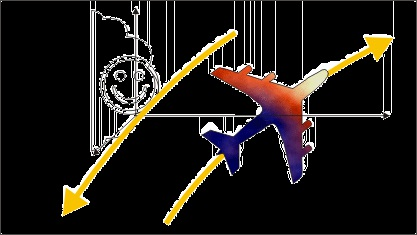Figure 1.3. Measurement from a moving reference

Take the case of observations about the motion of an aircraft made by two observers one at a

ground and another attached to the cloud moving at certain speed. For the observer on the ground,

the aircraft is moving at a speed of ,say, 1000 km/hr.

Further, let the reference system attached to the cloud itself is moving, say, at the speed of 50

km/hr, in a direction opposite to that of the aircraft as seen by the person on the ground. Now,

locating ourselves in the frame of reference of the cloud, we can visualize that the aircraft is

changing its position more rapidly than as observed by the observer on the ground i.e. at the

combined speed and would be seen flying by the observer on the cloud at the speed of 1000 + 50

= 1050 km/hr.

We need to change our mind set

The scientific measurement requires that we change our mindset about perceiving motion and its

scientific meaning. To our trained mind, it is difficult to accept that a stationary building standing

at a place for the last 20 years is actually moving for an observer, who is moving towards it. Going

by the definition of motion, the position of the building in the coordinate system of an

approaching observer is changing with time. Actually, the building is moving for all moving

bodies. What it means that the study of motion requires a new scientific approach about

perceiving motion. It also means that the scientific meaning of motion is not limited to its

interpretation from the perspective of earth or an observer attached to it.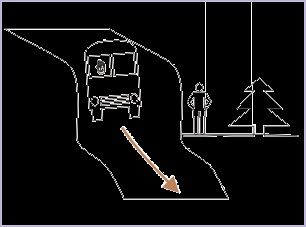Figure 1.4. Motion of a tree

Motion of the tree as seen by the person driving the truck

Consider the motion of a tree as seen from a person driving a truck (See Figure above) . The tree is undeniably stationary for a person standing on the ground. The coordinates of the tree in the

frame of reference attached to the truck, however, is changing with time. As the truck moves

ahead, the coordinates of the tree is increasing in the opposite direction to that of the truck. The

tree, thus, is moving backwards for the truck driver – though we may find it hard to believe as the

tree has not changed its position on the ground and is stationary. This deep rooted perception

negating scientific hard fact is the outcome of our conventional mindset based on our life long

perception of the bodies grounded to the earth.

Is there an absolute frame of reference?

Let us consider following :

In nature, we find that smaller entities are contained within bigger entities, which themselves are

moving. For example, a passenger is part of a train, which in turn is part of the earth, which in turn

is part of the solar system and so on. This aspect of containership of an object in another moving

object is chained from smaller to bigger bodies. We simply do not know which one of these is the

ultimate container and the one, which is not moving.

These aspects of motion as described in the above paragraph leads to the following conclusions

"There is no such thing like a “mother of all frames of reference” or the ultimate container, which

can be considered at rest. As such, no measurement of motion can be considered absolute. All

measurements of motion are, therefore, relative."

Motion types

Nature displays motions of many types. Bodies move in a truly complex manner. Real time

motion is mostly complex as bodies are subjected to various forces. These motions are not simple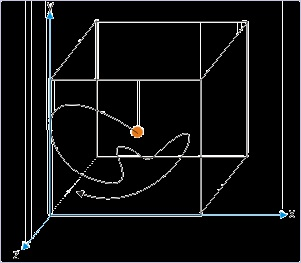straight line motions. Consider a bird’s flight for example. Its motion is neither in the straight line

nor in a plane. The bird flies in a three dimensional space with all sorts of variations involving

direction and speed. A boat crossing a river, on the other hand, roughly moves in the plane of

water surface.

Motion in one dimension is rare. This is surprising, because the natural tendency of all bodies is to

maintain its motion in both magnitude and direction. This is what Newton’s first law of motion

tells us. Logically all bodies should move along a straight line at a constant speed unless it is

acted upon by an external force. The fact of life is that objects are subjected to verities of forces

during their motion and hence either they deviate from straight line motion or change speed.

Since, real time bodies are mostly non-linear or varying in speed or varying in both speed and

direction, we may conclude that bodies are always acted upon by some force. The most common

and omnipresent forces in our daily life are the gravitation and friction (electrical) forces. Since

force is not the subject of discussion here, we shall skip any further elaboration on the role of

force. But, the point is made : bodies generally move in complex manner as they are subjected to

different forces.

Figure 1.5. Real time motion

A gas molecule in a container moves randomly under electrostatic interaction with other molecules

Nevertheless, study of motion in one dimension is basic to the understanding of more complex

scenarios of motion. The very nature of physical laws relating to motion allows us to study motion

by treating motions in different directions separately and then combining the motions in

accordance with vector rules to get the overall picture.

A general classification of motion is done in the context of the dimensions of the motion. A

motion in space, comprising of three dimensions, is called three dimensional motion. In this case,

all three coordinates are changing as the time passes by. While, in two dimensional motion, any

two of the three dimensions of the position are changing with time. The parabolic path described

by a ball thrown at certain angle to the horizon is an example of the two dimensional motion (See

Figure). A ball thrown at an angle with horizon is described in terms of two coordinates x and y.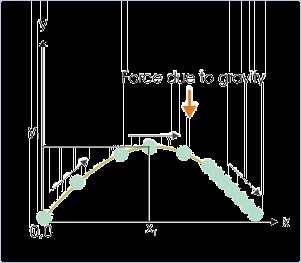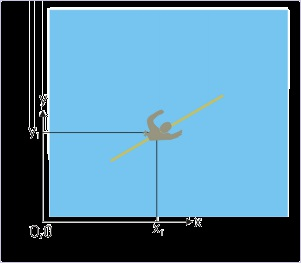Figure 1.6. Two dimensional motion

A ball thrown at an angle with horizon is described in terms of two coordinates x and y

One dimensional motion, on the other hand, is described using any one of the three coordinates;

remaining two coordinates remain constant through out the motion. Generally, we believe that one

dimensional motion is equivalent to linear motion. This is not further from truth either. A linear

motion in a given frame of reference, however, need not always be one dimensional. Consider the

motion of a person swimming along a straight line on a calm water surface. Note here that

position of the person at any given instant in the coordinate system is actually given by a pair of

coordinate (x,y) values (See Figure below).

Figure 1.7. Linear motion

Description of motion requires two coordinate values

There is a caveat though. We can always rotate the pair of axes such that one of it lies parallel to

the path of motion as shown in the figure. One of the coordinates, y y1 is constant through out the

motion. Only the x-coordinate is changing and as such motion can be described in terms of x-

coordinate alone. It follows then that all linear motion can essentially be treated as one

dimensional motion.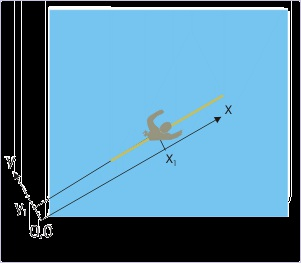Figure 1.8. Linear and one dimensional motion

Choice of appropriate coordinate system renders linear motion as one dimensional motion.

Kinematics

Kinematics refers to the study of motion of natural bodies. The bodies that we see and deal with in

real life are three dimensional objects and essentially not a point object.

A point object would occupy a point (without any dimension) in space. The real bodies, on the

other hand, are entities with dimensions, having length, breadth and height. This introduces

certain amount of complexity in so far as describing motion. First of all, a real body can not be

specified by a single set of coordinates. This is one aspect of the problem. The second equally

important aspect is that different parts of the bodies may have path trajectories different to each

other.

When a body moves with rotation (rolling while moving), the path trajectories of different parts

of the bodies are different; on the other hand, when the body moves without rotation (slipping/

sliding), the path trajectories of the different parts of the bodies are parallel to each other.

In the second case, the motion of all points within the body is equivalent as far as translational

motion of the body is concerned and hence, such bodies may be said to move like a point object. It

is, therefore, possible to treat the body under consideration to be equivalent to a point so long

rotation is not involved.

For this reason, study of kinematics consists of studies of :

1. Translational kinematics

2. Rotational kinematics

A motion can be pure translational or pure rotational or a combination of the two types of motion.

The translational motion allows us to treat a real time body as a point object. Hence, we freely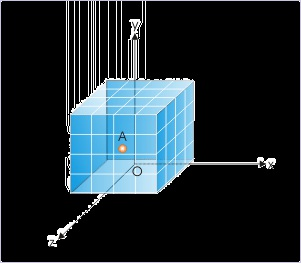refer to bodies, objects and particles in one and the same sense that all of them are point entities,

whose position can be represented by a single set of coordinates. We should keep this in mind

while studying translational motion of a body and treating the same as point.

1.2. Coordinate systems in physics*

Coordinate system is a system of measurement of distance and direction with respect to rigid

bodies. Structurally, it comprises of coordinates and a reference point, usually the origin of the

coordinate system. The coordinates primarily serve the purpose of reference for the direction of

motion, while origin serves the purpose of reference for the magnitude of motion.

Measurements of magnitude and direction allow us to locate a position of a point in terms of

measurable quantities like linear distances or angles or their combinations. With these

measurements, it is possible to locate a point in the spatial extent of the coordinate system. The

point may lie anywhere in the spatial (volumetric) extent defined by the rectangular axes as shown

in the figure. (Note : The point, in the figure, is shown as small sphere for visual emphasis only)

Figure 1.9. A point in the coordinate system

A distance in the coordinate system is measured with a standard rigid linear length like that of a

“meter” or a “foot”. A distance of 5 meters, for example, is 5 times the length of the standard

length of a meter. On the other hand, an angle is defined as a ratio of lengths and is dimensional-

less. Hence, measurement of direction is indirectly equivalent to the measurement of distances

only.

The coordinate system represents the system of rigid body like earth, which is embodied by an

observer, making measurements. Since measurements are implemented by the observer, they (the

measurements in the coordinate system) represent distance and direction as seen by the observer.

It is, therefore, clearly implied that measurements in the coordinates system are specific to the

state of motion of the coordinate system.

In a plane language, we can say that the description of motion is specific to a system of rigid

bodies, which involves measurement of distance and direction. The measurements are done, using

standards of length, by an observer, who is at rest with the system of rigid bodies. The observer

makes use of a coordinate system attached to the system of rigid bodies and uses the same as

reference to make measurements.

It is apparent that the terms “system of rigid bodies”, “observer” and “coordinate system” etc. are

similar in meaning; all of which conveys a system of reference for carrying out measurements to

describe motion. We sum up the discussion thus far as :

1. Measurements of distance, direction and location in a coordinate system are specific to the

system of rigid bodies, which serve as reference for both magnitude and direction.

2. Like point, distance and other aspects of motion, the concept of space is specific to the

reference represented by coordinate system. It is, therefore, suggested that use of word “space”

independent of coordinate system should be avoided and if used it must be kept in mind that it

represents volumetric extent of a specific coordinate system. The concept of space, if used

without caution, leads to an inaccurate understanding of the laws of nature.

3. Once the meanings of terms are clear, “the system of reference” or “frame of reference” or

“rigid body system” or “observer” or “coordinate system” may be used interchangeably to

denote an unique system for determination of motional quantities and the representation of a

motion.

Coordinate system types

Coordinate system types determine position of a point with measurements of distance or angle or

combination of them. A spatial point requires three measurements in each of these coordinate

types. It must, however, be noted that the descriptions of a point in any of these systems are

equivalent. Different coordinate types are mere convenience of appropriateness for a given

situation. Three major coordinate systems used in the study of physics are :

Rectangular (Cartesian)

Spherical

Cylindrical

Rectangular (Cartesian) coordinate system is the most convenient as it is easy to visualize and

associate with our perception of motion in daily life. Spherical and cylindrical systems are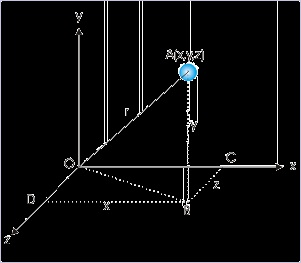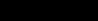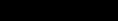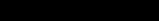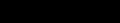specifically designed to describe motions, which follow spherical or cylindrical curvatures.

Rectangular (Cartesian) coordinate system

The measurements of distances along three mutually perpendicular directions, designated as x,y

and z, completely define a point A (x,y,z).

Figure 1.10. A point in rectangular coordinate system

A point is specified with three coordinate values

From geometric consideration of triangle OAB,

From geometric consideration of triangle OBD,

Combining above two relations, we have :

The numbers are assigned to a point in the sequence x, y, z as shown for the points A and B.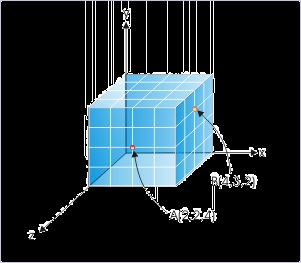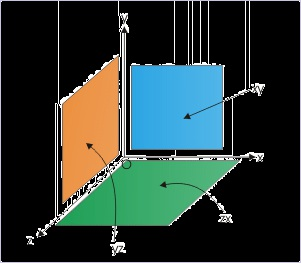Figure 1.11. Specifying points in rectangular coordinate system

A point is specified with coordinate values

Rectangular coordinate system can also be viewed as volume composed of three rectangular

surfaces. The three surfaces are designated as a pair of axial designations like “xy” plane. We may

infer that the “xy” plane is defined by two lines (x and y axes) at right angle. Thus, there are “xy”,

“yz” and “zx” rectangular planes that make up the space (volumetric extent) of the coordinate

system (See figure).

Figure 1.12. Planes in rectangular coordinate system

Three mutually perpendicular planes define domain of rectangular system

The motion need not be extended in all three directions, but may be limited to two or one

dimensions. A circular motion, for example, can be represented in any of the three planes,

whereby only two axes with an origin will be required to describe motion. A linear motion, on the

other hand, will require representation in one dimension only.

Spherical coordinate system

A three dimensional point “A” in spherical coordinate system is considered to be located on a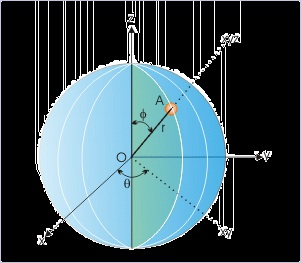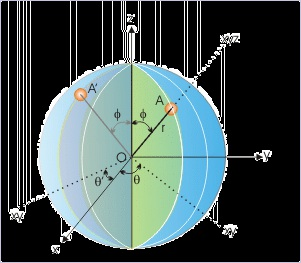sphere of a radius “r”. The point lies on a particular cross section (or plane) containing origin of

the coordinate system. This cross section makes an angle “θ” from the “zx” plane (also known as

longitude angle). Once the plane is identified, the angle, φ, that the line joining origin O to the

point A, makes with “z” axis, uniquely defines the point A (r, θ, φ).

Figure 1.13. Spherical coordinate system

A point is specified with three coordinate values

It must be realized here that we need to designate three values r, θ and φ to uniquely define the

point A. If we do not specify θ, the point could then lie any of the infinite numbers of possible

cross section through the sphere like A'(See Figure below).

Figure 1.14. Spherical coordinate system

A point is specified with three coordinate values

From geometric consideration of spherical coordinate system :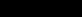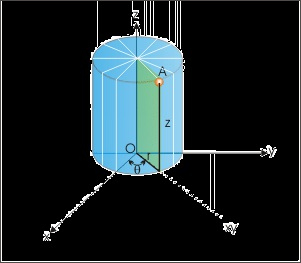These relations can be easily obtained, if we know to determine projection of a directional

quantity like position vector. For example, the projection of "r" in "xy" plane is "r sinφ". In turn, projection of "r sinφ" along x-axis is ""r sinφ cosθ". Hence,

In the similar fashion, we can determine other relations.

Cylindrical coordinate system

A three dimensional point “A” in cylindrical coordinate system is considered to be located on a

cylinder of a radius “r”. The point lies on a particular cross section (or plane) containing origin of

the coordinate system. This cross section makes an angle “θ” from the “zx” plane. Once the plane

is identified, the height, z, parallel to vertical axis “z” uniquely defines the point A(r, θ, z)

Figure 1.15. Cylindrical coordinate system

A point is specified with three coordinate values

1.3. Distance*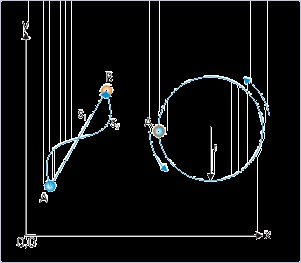1.3. Distance*

Distance represents the magnitude of motion in terms of the "length" of the path, covered by an

object during its motion. The terms "distance" and "distance covered" are interchangeably used to

represent the same length along the path of motion and are considered equivalent terms. Initial

and final positions of the object are mere start and end points of measurement and are not

sufficient to determine distance. It must be understood that the distance is measured by the length

covered, which may not necessarily be along the straight line joining initial and final positions.

The path of the motion between two positions is an important consideration for determining

distance. One of the paths between two points is the shortest path, which may or may not be

followed during the motion.

Definition: Distance

Distance is the length of path followed during a motion.

Figure 1.16. Distance

Distance depends on the choice of path between two points

In the diagram shown above, s 1 , represents the shortest distance between points A and B.

Evidently,

s 2 ≥ s 1

The concept of distance is associated with the magnitude of movement of an object during the

motion. It does not matter if the object goes further away or suddenly moves in a different

direction or reverses its path. The magnitude of movement keeps adding up so long the object

moves. This notion of distance implies that distance is not linked with any directional attribute.

The distance is, thus, a scalar quantity of motion, which is cumulative in nature.

An object may even return to its original position over a period of time without any “net” change

in position; the distance, however, will not be zero. To understand this aspect of distance, let us

consider a point object that follows a circular path starting from point A and returns to the initial

position as shown in the figure above. Though, there is no change in the position over the period

of motion; but the object, in the meantime, covers a circular path, whose length is equal to its

perimeter i.e. 2πr.

Generally, we choose the symbol 's' to denote distance. A distance is also represented in the form

of “∆s” as the distance covered in a given time interval ∆t. The symbol “∆” pronounced as “del”

signifies the change in the quantity before which it appears.

Distance is a scalar quantity but with a special feature. It does not take negative value unlike some

other scalar quantities like “charge”, which can assume both positive and negative values. The

very fact that the distance keeps increasing regardless of the direction, implies that distance for a

body in motion is always positive. Mathematically : s > 0

Since distance is the measurement of length, its dimensional formula is [L] and its SI

measurement unit is “meter”.

Distance – time plot

Distance – time plot is a simple plot of two scalar quantities along two axes. However, the nature

of distance imposes certain restrictions, which characterize "distance - time" plot.

The nature of "distance – time" plot, with reference to its characteristics, is summarized here :

1. Distance is a positive scalar quantity. As such, "the distance – time" plot is a curve in the first

quadrant of the two dimensional plot.

2. As distance keeps increasing during a motion, the slope of the curve is always positive.

3. When the object undergoing motion stops, then the plot becomes straight line parallel to time

axis so that distance is constant as shown in the figure here.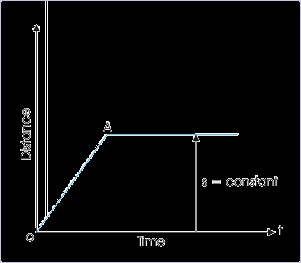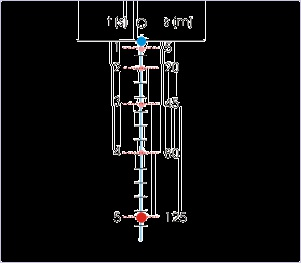Figure 1.17. Distance - time plot

One important implication of the positive slope of the "distance - time" plot is that the curve never

drops below a level at any moment of time. Besides, it must be noted that "distance - time" plot is

handy in determining "instantaneous speed", but we choose to conclude the discussion of "distance

- time" plot as these aspects are separately covered in subsequent module.

Example 1.1. Distance – time plot

Question : A ball falling from an height ‘h’ strikes the ground. The distance covered during

the fall at the end of each second is shown in the figure for the first 5 seconds. Draw distance –

time plot for the motion during this period. Also, discuss the nature of the curve.

Figure 1.18. Motion of a falling ball

Solution : We have experienced that a free falling object falls with increasing speed under the

influence of gravity. The distance covered in successive time intervals increases with time.

The magnitudes of distance covered in successive seconds given in the plot illustrate this

point. In the plot between distance and time as shown, the origin of the reference (coordinate

system) is chosen to coincide with initial point of the motion.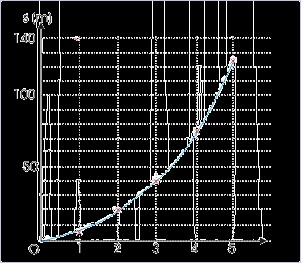Figure 1.19. Distance – time plot

From the plot, it is clear that the ball covers more distance as it nears the ground. The

"distance- time" curve during fall is, thus, flatter near start point and steeper near earth

surface. Can you guess the nature of plot when a ball is thrown up against gravity?

Exercise 1.

A ball falling from an height ‘h’ strikes a hard horizontal surface with increasing speed. On each

rebound, the height reached by the ball is half of the height it fell from. Draw "distance – time"

plot for the motion covering two consecutive strikes, emphasizing the nature of curve (ignore

actual calculation). Also determine the total distance covered during the motion.

Here we first estimate the manner in which distance is covered under gravity as the ball falls or

rises.

The distance- time curve during fall is flatter near start point and steeper near earth surface. On

the other hand, we can estimate that the distance- time curve, during rise, is steeper near the earth

surface (covers more distance due to greater speed) and flatter as it reaches the maximum height,

when speed of the ball becomes zero.

The "distance – time" plot of the motion of the ball, showing the nature of curve during motion, is

: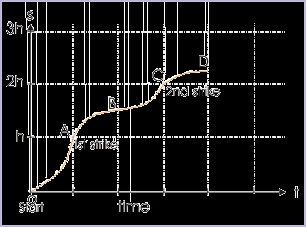Figure 1.19. Distance – time plot

The origin of plot (O) coincides with the initial position of the ball (t = 0). Before striking the

surface for the first time (A), it travels a distance of ‘h’. On rebound, it rises to a height of ‘h/2’

(B on plot). Total distance is ‘h + h/2 = 3h/2’. Again falling from a height of ‘h/2’, it strikes the

surface, covering a distance of ‘h/2’. The total distance from the start to the second strike (C on

plot) is ‘3h/2 + h/2 = 2h’.

1.4. Position*

Coordinate system enables us to specify a point in its defined volumetric space. We must

recognize that a point is a concept without dimensions; whereas the objects or bodies under

motion themselves are not points. The real bodies, however, approximates a point in translational